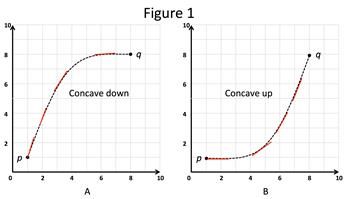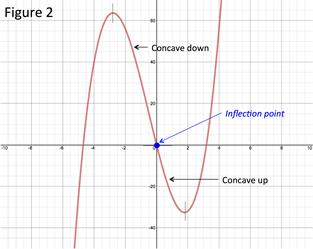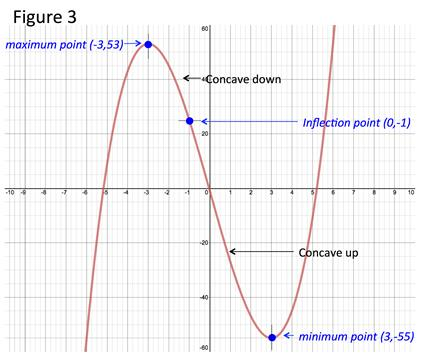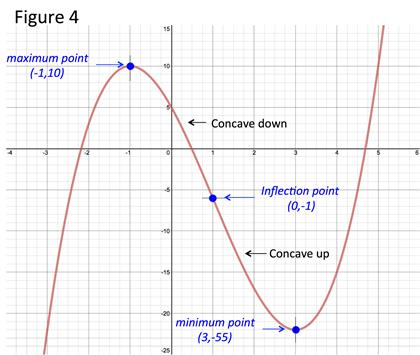# The Second Derivative Rule

The second derivative can be used to determine the concavity and inflection point of a function as well as minimum and maximum points.

Figure 1 shows two graphs that start and end at the same points but are not the same.In Graph A the slope of the tangent lines are decreasing from p to q where q > p and is said to be concave down. In Graph B the slope of the tangent lines are decreasing from p to q where q > p and is said to be concave up. The change of the slopes of the tangent lines is the second derivative of the function. Hence we have a test for concavity using the second derivative.

If the second derivative is negative over an interval, indicating that the change of the slope of the tangent line is decreasing, the graph is concave down over that interval.

If the second derivative is positive over an interval, indicating that the change of the slope of the tangent line is increasing, the graph is concave up over that interval.

CONCAVITY TEST:

If f ''(x) < 0 over an interval, then the graph of f is concave upward over this interval.

If f ''(x) > 0 over an interval, then the graph of f is concave downward over this interval.

The second derivative can also reveal the point of inflection. The point where a graph changes between concave up and concave down is called an inflection point, See Figure 2.If the second derivative is positive/negative on one side of a point and the opposite sign on the other side, the point is an inflection point.

INFLECTION POINT TEST:

Let a, b and c be points on a graph such that a > b > c.

• If f '' > 0, from a to b and f(x) < 0 from b to c there is an inflection point at b.

• If f '' < 0, from a to b and f(x) > 0 from b to c there is an inflection point at b

As a result of the concavity test, the second derivative can also be used to reveal minimum and maximum points.

If the first derivative of a point is zero it is a local minimum or a local maximum, See First Derivative Test.

If the second derivative of that same point is positive the point is a local minimum. If the second derivative of that same point is negative, the point is a local maximum. This is called the Second Derivative Test.

SECOND DERIVATIVE TEST - LOCAL MINIMUMS AND MAXIMUMS:

Let f '' is continuous near c.

• If f '' (c) > 0, there is a local minimum at c.

• If f '' (c) < 0, there is a local maximum at c.

Let's look at some examples.

To work these examples requires the use of various derivative rules. If you are not familiar with a rule go to the associated topic for a review.

Example 1: Determine the intervals of concavity, minimum/maximum points and inflection points and sketch the graph for f(x) = x3 - 27x - 1.

Step 1: Determine the values of x when the second derivative equals 0.

Find the second derivative:

f(x) = x3 - 27x - 1

${f}^{\prime }\left(x\right)=3{x}^{2}-27$

${f}^{″}\left(x\right)=6x$

Set the second derivative equal to zero:

0 = 6x

Set each factor to zero and solve:

0 = 6x

x = 0

Step 2: Determine concavity.

Create a table of intervals that end/begin with x-values such that $f\text{'}\text{'}\left(x\right)=0$.

To determine the sign of the second derivative select a number in the interval and solve.

If the second derivative on an interval is positive, the function is concave up over that interval. If the second derivative on an interval is negative, the function is concave down over that interval.

 Interval ${f}^{″}\left(x\right)=6x$ Concavity $-\infty \le x\le 0$ ${f}^{″}\left(-4\right)=6\left(-4\right)=-24$ - Concave down $0\le x\le \infty$ ${f}^{″}\left(1\right)=6\left(1\right)=6$ + Concave up

Step 3: Determine the Inflection Point.

From the table the second derivative changes from negative to positive at 0 thus there is an inflection point at zero. The coordinates of the inflection point are:

$f\left(0\right)={\left(0\right)}^{3}-27\left(0\right)-1=-1;\left(0,-1\right)$

Step 4: Apply the Second Derivative Test to determine the maximum/minimum points.

Determine the x-values when ${f}^{\prime }=0$

${f}^{\prime }\left(x\right)=3{x}^{2}-27$ from Step 1

0 = 3x2 - 27    Set to 0

0 = 3(x - 3)(x + 3) Factor

$3\ne 0$

$x-3=0\to x=3$

$x+3=0\to x=-3$ Solve for x

Determine the value of $f\text{'}\text{'}$ of the x-values when ${f}^{\prime }=0$

${f}^{″}\left(3\right)=6\left(x\right)=18$

${f}^{″}\left(-3\right)=6\left(-3\right)=-18$

Hence there is a minimum point at 3 and a maximum point at -3. The coordinates of these points are:

$f\left(3\right)={3}^{3}-27\left(3\right)-1=-55\to \left(3,-55\right)$     Local minimum

Local maximum

Step 5: Sketch GraphExample 2: Determine the intervals of concavity, minimum/maximum points and inflection points and sketch the graph for f(x) = (x - 1)3 - 12x + 6.

Step 1: Determine the values of x when the second derivative equals 0.

Find the second derivative:

f(x) = (x - 1)3 - 12x + 6

${f}^{\prime }\left(x\right)=3{\left(x-1\right)}^{2}-12$

${f}^{″}\left(x\right)=6\left(x-1\right)$

Set the second derivative equal to zero:

0 = 6(x - 1)

Set each factor to zero and solve:

$0\ne 6$

Step 2: Determine concavity.

Create a table of intervals that end/begin with x-values such that $f\text{'}\text{'}\left(x\right)=0$.

To determine the sign of the second derivative select a number in the interval and solve.

If the second derivative on an interval is positive, the function is concave up over that interval. If the second derivative on an interval is negative, the function is concave down over that interval.

 Interval ${f}^{″}\left(x\right)=6x$ Concavity $-\infty \le x\le 1$ ${f}^{″}\left(-2\right)=6\left(-2-1\right)=-18$ - Concave down $1\le x\le \infty$ ${f}^{″}\left(2\right)=6\left(2-1\right)=6$ + Concave up

Step 3: Determine the Inflection Point.

From the table the second derivative changes from negative to positive at 1 thus there is an inflection point at 1. The coordinates of the inflection point are:

Step 4: Apply the Second Derivative Test to determine the maximum/minimum points.

Determine the x-values when ${f}^{\prime }=0$

${f}^{\prime }\left(x\right)=3{\left(x-1\right)}^{2}-12$ from Step 1

0 = 3(x - 1)2 - 12  Set to 0

12 = 3(x - 1)2

4 = (x - 1)2

$±2=x-1$

Solve for x

Determine the value of $f\text{'}\text{'}$ of the x-values when ${f}^{\prime }=0$

${f}^{″}\left(3\right)=6\left(3-1\right)=12$

${f}^{″}\left(-1\right)=6\left(-1-1\right)=-12$

Hence there is a minimum point at 3 and a maximum point at -1. The coordinates of these points are:

$f\left(3\right)={\left(3-1\right)}^{3}-12\left(3\right)+6=-22\to \left(3,-22\right)$ Local minimum

$f\left(-1\right)={\left(-1-1\right)}^{3}-12\left(-1\right)+6=10\to \left(-1,10\right)$     Local maximum

Step 5: Sketch Graph